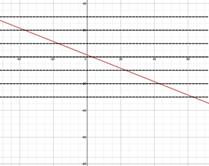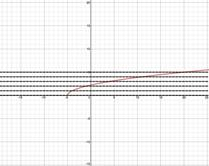# Inverse Functions: Finding Inverse Functions Analytically

Finding an inverse function for f(x) = x - 1 is easily done by inspection, f -1(x) = x + 1. However for more complicated functions follow these guidelines.

DEFINITION OF ONE-TO-ONE:

• Graph the function and apply the Horizontal Line Test to determine if the function is one-to-one and thus has an inverse function.

• Take the function equation and replace f(x) by y.

• Switch the x and the y in the function equation and solve for y.

• Replace y by f -1(x).

• Verify that f(x) and f -1(x) are inverse functions.

º Show that f[f -1(x)] = x.
º Show that f[f -1(x)] = x.
º Show that the domain and range have been reversed.

Here is a review of the Horizontal Line Test.

HORIZONTAL LINE TEST:

A function f is one-to-one and has an inverse function if and only if no horizontal line intersects the graph of f at more than one point.

Let's use these guidelines to determine the inverse of a function.

Example 1: Find the inverse function for $f\left(x\right)=\frac{3-x}{2}$.

Step 1: Graph the function and apply the Horizontal Line Test to determine if the function is one-to-one and thus has an inverse function.

No horizontal line intersects the graph in more than one place. Thus the function is one-to-one and has an inverse function.Step 2: Take the function equation and replace f(x) by y.

$f\left(x\right)=\frac{3-x}{2}$

$y=\frac{3-x}{2}$

Step 3: Switch the x and y in the function equation and solve for y.

$y=\frac{3-x}{2}$ Original

$x=\frac{3-y}{2}$  Switch x and y

2x = 3 - y   Multiply by 2

y + 2x = 3  Add y

y = 3 - 2x   Subtract 2x

Step 4: Replace y by f -1(x).

y = 3 - 2x

f -1(x) = 3 - 2x

Step 5: Verify that f(x) and f -1(x) are inverse functions.

1. Show that f[f -1(x)] = x.

2. Show that f -1[f(x)] = x.

3. Show that the domain and range have been reversed.

1. $f\left[{f}^{-1}\left(x\right)\right]=f\left(3-2x\right)=\frac{3-\left(3-2x\right)}{2}=\frac{3-3+2x}{2}=\frac{2x}{2}=x$

2. ${f}^{-1}\left[f\left(x\right)\right]={f}^{-1}\left(\frac{3-x}{2}\right)=3-2\left(\frac{3-x}{2}\right)=3-\left(3-x\right)=3-3+x=x$

3.

 x $f\left(x\right)=\frac{3-x}{2}$ x ${f}^{-1}\left(x\right)=3-2x$ 0 1.5 1.5 0 1 1 1 1 2 0.5 0.5 2 3 0 0 3

Verified, the inverse function of $f\left(x\right)=\frac{3-x}{2}$ is ${f}^{-1}\left(x\right)=3-2x$

Example 2: Find the inverse function for $f\left(x\right)=\sqrt{x+5}$.

Step 1: Graph the function and apply the Horizontal Line Test to determine if the function is one-to-one and thus has an inverse function.

No horizontal line intersects the graph in more than one place. Thus the function is one-to-one and has an inverse function.Step 2: Take the function equation and replace f(x) by y.

$f\left(x\right)=\sqrt{x+5}$

$y=\sqrt{x+5}$

Step 3: Switch the x and y in the function equation and solve for y.

$y=\sqrt{x+5}$ Original

$x=\sqrt{y+5}$ Switch x and y

${x}^{2}=y+5$   Square

${x}^{2}-5=y$   Subtract 5

Step 4: Replace y by f -1(x).

$y={x}^{2}-5$

${f}^{-1}\left(x\right)={x}^{2}-5$

Step 5: Verify that f(x) and f -1(x) are inverse functions.

4. Show that f[f -1(x)] = x.

5. Show that f -1[f(x)] = x.

6. Show that the domain and range have been reversed.

1. $f\left[{f}^{-1}\left(x\right)\right]=f\left({x}^{2}-5\right)=\sqrt{\left({x}^{2}-5\right)+5}=\sqrt{{x}^{2}}=x$

2. ${f}^{-1}\left[f\left(x\right)\right]={f}^{-1}\left(\sqrt{x+5}\right)={\left(\sqrt{x+5}\right)}^{2}-5=\left(x+5\right)-5=x$

3.

 x $f\left(x\right)=\sqrt{x+5}$ x ${f}^{-1}\left(x\right)={x}^{2}-5$ 0 $\sqrt{5}$ $\sqrt{5}$ 0 1 $\sqrt{6}$ $\sqrt{6}$ 1 2 $\sqrt{7}$ $\sqrt{7}$ 2 3 $\sqrt{8}$ $\sqrt{8}$ 3

Verified, the inverse function of $f\left(x\right)=\sqrt{x+5}$ is ${f}^{-1}\left(x\right)={x}^{2}-5$

 Related Links: Math algebra Conics: Classifying from General Equation Component Form and Magnitude Pre Calculus

To link to this Inverse Functions: Finding Inverse Functions Analytically page, copy the following code to your site: